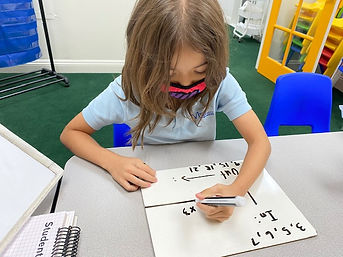Ms. Dawn

Target 1​

Lesson Type:

New

Number Operation

:

Number System

Use understandings of positive and negative number order to determine the value of a given space on a number line.

1:

Understand that a number line is a spatial representation of quantities.

2:

Understand that numbers have an unchanging order, so even if a number is not written on the number line (it still has a space).

3:

Indicate an integer on the number line.

4th

Vocabulary:

Integer, Positive number, Negative number, Number line

Activities:

1. Students numbered a blank number line on the board from positive 5 to negative 5.

2. When asked, students were able to indicate an integer on the number line.

3. Students created a number line by cutting out colored boxes that represented postive numbers, negative numbers, and zero. Then, they needed to place colored stars on certain integers.Home Exploration

Guiding Questions:Absent Students:

Target 2

:

1:

Understand how to use place-value (the value of each digit) to compare numbers.

2:

Compare integers (positive and negative numbers).

3:

Order a set of integers from least to greatest (and greatest to least).

4th

Vocabulary:

Integer, Positive number, Negative number, Number line, Least, Greatest, Biggest, Smallest, Value

Activities:

1. Had a discussion about place value and how it applies to positive and negative integers.

2. Students compared positive and negative integers using playing cards. The black suit cards represented negative numbers and the red suit cards represented positive numbers. Students played a game exactly like "War." They flipped each card over and the player with the larger number took both cards.

3. Given a number line and few positive and negative integers on a whiteboard, students indicated which integer was the smallest and which one was the biggest.

4. Students used playing cards to order integers from least to greatest. The black suit cards represented negative integers and red suit cards represented positive integers.Home Exploration

Guiding Questions:Target 3

:

1:

Understand that a function is a rule that gives one output to each input.

2:

Understand that by studying the proportional relationship between inputs and outputs, a function (equation) can be derived.

4th

Vocabulary:

Input, Output, Rule, Function, Equation

Activities:

1. Given an input and output visual with numbers above the input and numbers by the output, students were able to identify the rule.

2. Students could look at an input/output visual and write an equation that identified the rule.Home Exploration